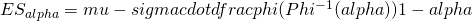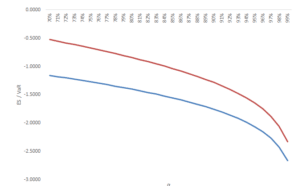# Expected shortfall closed form

The expected shortfall (ES), also called the conditional value-at-risk, is a tail-risk measure used to accommodate some shortcomings of VaR. The expected shortfall calculates the expected return (loss) based on the x% worst occurrences. As such, it relationship towards VaR becomes more clear. Specifically, the VaR tells you that the loss will not be greater than a certain amount over a certain period with x% probability. The expected shortfall tells you what to average loss will be over a certain period given the VaR has been breached.

## Expected shortfall formula

An alternative to calculating the ES using empirical data or simulated data, is by assuming an underlying distribution. By for example assuming an underlying normal distribution, it is possible to calculate the ES using a closed form solution. This procedure has as advantages that it avoids the collection, processing and simulation of large amounts of data. Moreover, a closed form approach also avoids any sampling errors that are present in empirical, and simulation approaches. The formula below states the expected loss for the 1-α worst cases.The figure below compares the ES at various percentiles for a normal distribution with mean equal to 0 and standard deviation of 1. Notice that the ES is always smaller than the VaR. This is because the expected shortfall gives a feeling how bet things can get in case the losses exceed the VaR.## Expected shortfall intuition

As with the expected shortfall calculated from empirical data, it has as benefit to counter possible manipulation on the use of VaR. This is because the VaR only measures the amount at risk up to a certain percentile. Therefore risk could be intentionally or unintentionally be built up right after the VaR threshold without being observed at all. This could for example happen through an excessive amount of option-writing. Additionally, since assuming the normal distribution, the outcome is known, for a given mean, standard deviation and percentile. As such, the calculation is exposed to less manipulation possibilities. On the downside, the assumed distribution, may not be correct resulting in a wrong ES.

## Summary

The ES is a tail-risk measure developed to counter some shortcomings of VaR. It used used both for managing market and credit risk of investment portfolios and tells you what the expected loss is given the x% worst return occurrences in the portfolio.